﻿ 计及发电权交易和碳交易的含风电电力系统低碳经济调度研究 Study on Low Carbon Economic Dispatch of Wind Power System Considering Generation Rights Trading and Carbon Trading

Advances in Energy and Power Engineering
Vol.06 No.01(2018), Article ID:23835,13 pages
10.12677/AEPE.2018.61006

Study on Low Carbon Economic Dispatch of Wind Power System Considering Generation Rights Trading and Carbon Trading

Jialin Hu1, Cuimei Zhao2, Cheng Xiao3, Deyang Hu2, Jiaqing Zhong2

1China Electric Power Complete Equipment Co., Ltd., Beijing

2Hebei Provincial Key Laboratory of Power Electronics Energy Saving and Drive Control (Yanshan University), Qinhuangdao Hebei

3Tangshan City Fengrun District Power Supply Branch, State Grid North Hebei Electric Power Co., Ltd., Tangshan Hebei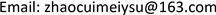Received: Feb. 6th, 2018; accepted: Feb. 20th, 2018; published: Feb. 27th, 2018ABSTRACT

Low carbon economy is the future direction of power dispatching, the introduction of wind power and other clean energy can ease fossil energy depletion, slow down environmental degradation, and promote the low-carbon development of the power industry. The output of wind power has the characteristics of intermittence and randomness. In view of this characteristic, the cost of wind farm surplus penalty and the reserve penalty cost are introduced into the cost of wind power, and the point effect cost and start stop cost are introduced into the generation cost of conventional unit. Power generation trading is a unique type of transaction in the power industry, and has the function of energy saving and emission reduction. Therefore, the power generation transaction is integrated into the power generation transaction in the carbon trading, taking into account the combined effects of carbon trading and power generation trading. In summary, this paper proposes a scheduling model of low carbon balance and economy, and the use of bacterial colony chemotaxis algorithm is used to verify the effectiveness of the new scheduling model.

Keywords:Carbon Trading, Power Generation Right Transaction, Wind Power, The Optimization Model Transaction Type

1中国电能成套设备有限公司，北京

2河北省电力电子节能与传动控制重点实验室(燕山大学)，河北 秦皇岛

3国网冀北电力有限公司唐山市丰润区供电分公司，河北 唐山1. 引言

2. 计及发电权交易和碳交易的含风电电力系统低碳经济调度模型

2.1. 系统在发电权交易和碳交易下成本最低的低碳调度目标

2.1.1. 基于发电权交易的调度模型

${F}_{g}={\varphi }^{g}\sum _{i=1}^{N}\left(\sum _{t=1}^{T}{P}_{Git}-{g}_{0i}\right)$ (1)

2.1.2. 基于碳交易的低碳调度目标

${E}_{G.q}=\sum _{t=1}^{T}\sum _{i=1}^{N}{P}_{Git}\left({\delta }_{i}-\eta \right)$ (2)

${E}_{W.q}=\sum _{t=1}^{T}\sum _{j=1}^{M}{P}_{Wjt}\left(0-\eta \right)$ (3)

$\mathrm{min}{F}_{c}=\mathrm{min}\left({F}_{G.c}+{F}_{W.c}\right)$ (4)

${F}_{G.c}={\varphi }_{c{o}_{2}}\mathrm{max}\left[{E}_{p}-{E}_{G.q},0\right]+{\gamma }_{c{o}_{2}}\mathrm{min}\left[{E}_{p}-{E}_{G.q},0\right]$ (5)

${F}_{W.c}={\gamma }_{c{o}_{2}}{E}_{W.q}$ (6)

$\mathrm{min}{E}_{p}=\mathrm{min}\sum _{t=1}^{T}\sum _{i=1}^{N}{C}_{i1}\left({P}_{Git}\right)$ (7)

$\mathrm{min}{F}_{1}=\mathrm{min}\left({F}_{g}+{F}_{c}\right)$ (8)

2.2. 系统总发电成本最低的经济调度目标

2.2.1. 计及阀点效应的常规机组发电成本

$\mathrm{min}{F}_{G}=\sum _{i=1}^{N}\sum _{t=1}^{T}\left[\left[{U}_{it}{C}_{{i}_{2}}\left({P}_{Git}\right)+{U}_{it}\left(1-{U}_{i\left(t-1\right)}\right){S}_{i}\right]+{Q}_{it}\right]$ (9)

${C}_{{i}_{2}}\left({P}_{Git}\right)={a}_{{i}_{2}}{P}_{Git}^{2}+{b}_{{i}_{2}}{P}_{Git}+{c}_{{i}_{2}}$ (10)

${Q}_{it}=|{e}_{i}\cdot \mathrm{sin}\left[{f}_{i}\cdot \left({P}_{i\mathrm{min}}-{P}_{Git}\right)\right]|$ (11)

2.2.2. 风电运行成本

· 风电运营成本

${F}_{W}=\sum _{j=1}^{M}{C}_{W}{P}_{Wjt}$ (12)

· 备用惩罚成本

${F}_{S}=\sum _{j=1}^{M}{C}_{S}\left(1-{\theta }_{jt}\right){P}_{sjt}$ (13)

· 盈余惩罚成本

${F}_{R}=\sum _{j=1}^{M}\left({C}_{W}-{C}_{R}\right)\left({P}_{sjt}-{P}_{Wjt}\right)$ (14)

$\mathrm{min}{F}_{2}=\mathrm{min}\sum _{t=1}^{T}\left({F}_{S}+{F}_{R}+{F}_{W}+{F}_{G}\right)$ (15)

2.3. 基于机会约束规划的低碳经济调度优化模型

2.3.1. 目标函数

$\mathrm{min}F=\mathrm{min}\left({F}_{1}+{F}_{2}\right)$ (16)

2.3.2. 约束条件

· 系统功率平衡约束。电力系统中发电厂的发电量必须满足系统的负荷才能保证电力系统正常运行。

${P}_{r}\left\{\sum _{i=1}^{N}{P}_{Git}+\sum _{j=1}^{M}{P}_{Wjt}-{P}_{Lt}\ge 0\right\}\ge {\beta }_{0}$ (17)

· 系统的正、负旋转备用约束。风电场接入系统后，系统需要增加正负旋转备用容量来应对风电出力的随机性对系统的影响。

${P}_{r}\left\{\sum _{i=1}^{N}{P}_{Gi}^{\mathrm{max}}+\sum _{j=1}^{M}{P}_{Wjt}\ge {P}_{Lt}\left(1+{R}_{u}\right)\right\}\ge {\beta }_{1}$ (18)

${P}_{r}\left\{\sum _{i=1}^{N}{P}_{Gi}^{\mathrm{min}}+\sum _{j=1}^{M}{P}_{Wjt}\le {P}_{Lt}\left(1-{R}_{d}\right)\right\}\ge {\beta }_{2}$ (19)

· 系统的爬坡能力的约束。系统的爬坡速率是系统单位时间内可以增加或减少出力。

$\left\{\begin{array}{l}{P}_{r}\left\{\sum _{i=1}^{N}{r}_{i}^{up}\ge \sum _{j=1}^{M}{\varphi }_{j}{P}_{Wjt}\right\}\ge {\beta }_{3}\\ {P}_{r}\left\{\sum _{i=1}^{N}{r}_{i}^{down}\ge \sum _{j=1}^{M}{\varphi }_{j}{P}_{Wjt}\right\}\ge {\beta }_{4}\end{array}$ (20)

· 常规火电机组出力约束。每台发电机组都应该满足自身的输出功率限制。

${P}_{Gi}^{\mathrm{min}}\le {P}_{Git}\le {P}_{Gi}^{\mathrm{max}}$ (21)

· 机组爬坡能力约束。发电机组的爬坡速率，每个机组单位时间内可以增加或减少出力。

$-{r}_{i}^{down}\Delta T\le {P}_{Git}-{P}_{Gi\left(t-1\right)}\le {r}_{i}^{up}\Delta T$ (22)

· 风电出力波动范围约束。风电功率的预测往往存在偏差，允许其出力上下偏差±25%，则风电场的随机出力范围满足以下约束条件  ：

$0.75{P}_{Wjt}\le {P}_{Wjt}\le 1.25{P}_{Wjt}$ (23)

· 风电穿透功率极限约束。

${P}_{Wjt}\le \mu {P}_{Lt}$ (24)

3. 模型求解方法

4. 算例分析

4.1. 初始数据

4.2. 优化调度结果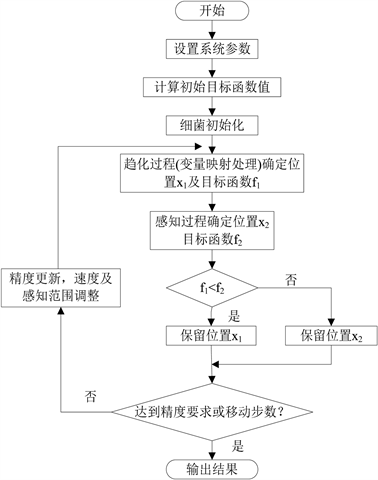Figure 1. Curve: BCC algorithm process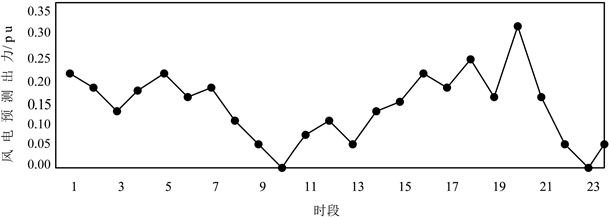Figure 2. Curve: The wind power predictionTable 3. System thermal power unit and the optimization of wind farm output results

4.3. 不同模型对比分析

1，当考虑发电权交易时为模式2。不同模式下各火电机组碳交易成本对比，如图3所示。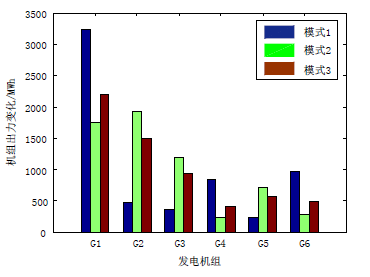Figure 3. Different mode of each thermal power unit cost of carbon trading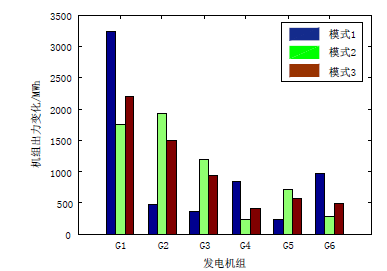Figure 4. Different mode of each thermal power unit carbon dioxide emissions

4.4. 碳交易价格对低碳经济调度模式的影响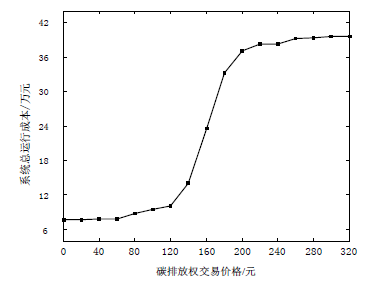Figure 5. System total operating costs of the carbon price change curve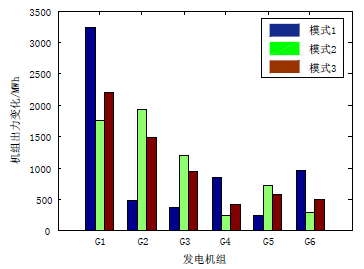Figure 6. Mode 2 when the average price and average conventional generators carbon trading cost comparison

5. 结论

Study on Low Carbon Economic Dispatch of Wind Power System Considering Generation Rights Trading and Carbon Trading[J]. 电力与能源进展, 2018, 06(01): 54-66. http://dx.doi.org/10.12677/AEPE.2018.61006

1. 1. 赵书强, 王扬, 徐岩, 殷加玞. 基于机会约束目标规划的高风电接入比例下大规模储能与火电协调调度[J]. 中国电机工程学报, 2016, 36(4): 969-977.

2. 2. 娄素华, 胡斌, 吴耀武, 卢斯煜. 碳交易环境下含大规模光伏电源的电力系统优化调度[J]. 电力系统自动化, 2014, 38(17): 91-97.

3. 3. 杨胡萍, 左士伟, 陈欢. 碳排放约束下考虑静态电压稳定的发电权交易优化[J]. 电网技术, 2014, 38(11): 3011-3015.

4. 4. Yang, M. (2007) China’s Energy Efficiency Target 2010. Energy Policy, 36, 561-570.
https://doi.org/10.1016/j.enpol.2007.09.032

5. 5. Steenhof, P.A. (2006) Decomposition of Electricity Demand in China’s Industrial Sector. Energy Economics, 28, 370-384.
https://doi.org/10.1016/j.eneco.2006.02.006

6. 6. 魏一鸣, 等. 中国可持续发展战略报告[M]. 北京: 科学出版社, 2006.

7. 7. 朱永胜, 王杰, 瞿博阳, Suganthan, P. N. 含风电场的多目标动态环境经济调度[J]. 电网技术, 2015, 39(5): 1315-1322.

8. 8. 别朝红, 胡国伟, 谢海鹏, 李更丰. 考虑需求响应的含风电电力系统的优化调度[J]. 电力系统自动化, 2014, 38(13): 115-159.

9. 9. 国家发展和改革委员会应对气候变化司. 关于公布2012年中国区域电网基准线排放因子的公告[EB/OL]. 2012.

10. 10. 陈道君, 龚庆武, 张茂林. 考虑能源环境效益的含风电场多目标优化调度[J]. 中国电机工程学报, 2011, 31(13): 10-17.

11. 11. 刘文学, 梁军, 贠志皓, 李正茂, 董晓明. 考虑节能减排的多目标模糊机会约束动态经济调度[J]. 电工技术学报, 2016, 31(1): 62-70.

12. 12. Streimikiene, D. and Roos, I. (2009) GHG Emission Trading Im-plications on Energy Sector in Baltic States. Renewable and Sustainable Energy Reviews, 13, 854-862.
https://doi.org/10.1016/j.rser.2008.02.005

13. 13. OECD/IEA. (2003) Policies to Reduce Greenhouse Gas Emissions in Industry. Successful Approaches and Lessons Learned, Workshop Report.

14. 14. Yajvender, P. and Ashwanikumar, V. (2012) Influence of Renewable, Promotion Policies on Power System Operation with Carton Emission Trading. Nirma University International Conference, 1-6.

15. 15. Bahmani-Firouzi, B., Farjah, E. and Seifi, A. (2013) A New Algorithm for combined Heat and Power Dynamic Economic Dispatch Considering Valve-Point Effects. Energy, 52, 320-332.
https://doi.org/10.1016/j.energy.2013.01.004

16. 16. Basu, M. (2005) A Simulated Annealing-Based Goal-Attainment Method for Economic Emission Load Dispatch of Fixed Head Hydrothermal Power Systems. Inter-national Journal of Electrical Power & Energy Systems, 27, 147-153.
https://doi.org/10.1016/j.ijepes.2004.09.004

17. 17. 王士柏, 韩学山, 杨明, 顾世龙. 计及不确定性的等备用边际效用约束的电力系统经济调度[J]. 中国电机工程学报, 2015, 35(5): 1143-1152.

18. 18. 刘晓, 艾欣, 彭谦. 计及需求响应的含风电场电力系统发电与碳排放权联合优化调度[J]. 电网技术, 2012, 36(1): 213-218.

19. 19. Cai, J., Ma, X., Li, Q., et al. (2009) A Multi-Objective Chaotic Particle Swarm Optimization for Environmental/Economic Dispatch. Energy Conversion and Management, 50, 1318-1325.
https://doi.org/10.1016/j.enconman.2009.01.013

20. 20. 傅利, 周步祥, 林虹江, 王小红. 基于混合变量动态优化算法的含风电电力系统多目标动态优化调度[J]. 电测与仪表, 2015, 52(10): 1-7.

21. 21. Chen, C.L. (2008) Optimal Wind-Thermal Generating Unit Commitment. IEEE Transactions on Energy Conversion, 23, 273-280.
https://doi.org/10.1109/TEC.2007.914188

22. 22. 张晓辉, 闫鹏达, 钟嘉庆, 卢志刚. 可再生能源激励制度下的低碳经济电源规划[J]. 电网技术, 2015, 39(3): 655-662.